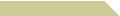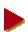# AMIN WITNO WEBPHILADELPHIA UNIVERSITYHOME COURSES NOTES EXAMS RÉSUMÉ

Input here >>

##How Do You Count?

Our counting system is set in base-10, also known as decimal (Greek: deca-), because we use 10 digits (0, 1, 2, 3, 4, 5, 6, 7, 8, and 9) to represent the integers. If we have only 0 and 1, the number system will be binary (Latin: bi-), and we count like this:

0, 1, 10, 11, 100, 101, 110, 111, 1000, 1001, 1010, 1011, …

Another system related to computing is base-16 (Greek: hexa-decimal), where the digits are 0 to 9, followed by A, B, C, D, E, and F. For example, just as the number 5827 in decimal actually represents the sum of 5 thousands (103), 8 hundreds (102), 2 tens (101), and 7 ones (100), so the hexadecimal number E2A9 represents the quantity 58025 (when written in decimal) as the following sum.

E2A9 = 14 × (163) + 2 × (162) + 10 × (161) + 9 × (160) = 58025

##Why 36?

Now if we extend this 16-digit idea by exhausting the entire English alphabet (26 letters from A to Z), then we get a system of counting in base-36 (0 to 9 plus A to Z).

We do not know if base-36 has a name, but we know that a base-6 counting system (employing only the digits 0 to 5) is called senary (Latin: sex-). Since 36 = 62, we can view base-36 as a senary with digital grouping of two, i.e., with a separating comma every other digit, e.g., we write the senary number 3550124 as 03,55,01,24. In all we are dealing with 36 possible pairs of digits (each digit being 0, 1, 2, 3, 4, or 5), ranging in decimal values from 0 to 35:

00, 01, 02, 03, 04, 05, 10, 11, 12, 13, 14, 15, 20, … , 54, 55

We then simplify: 00 = 0, 01 = 1, … , 54 = Y, 55 = Z, and omit the commas, e.g., 3550124 (base-6) is now written 3Z1G (base-36).

Thus the name bi-sen-a-ry, our own definition.

##What Is Your Number?

Hence every word can be treated as a bisenary number or be converted to a decimal value. For instance, we find that the name Ali is equivalent to 13734:

ALI = (10 × 362) + (21 × 36) + (18 × 1) = 13734

Your required contribution is to write a program to do the conversion between decimal and bisenary. Choose a programming language of your preference, making sure that from bisenary to decimal the user may input a lower-case digit (a) instead of upper-case (A).

To do the converse, from decimal to bisenary, you will deal with further mathematical reasoning. As a crude sample, this part has been implemented in JavaScript at the top of this page. Feel free to save a copy or modify this file as needed.